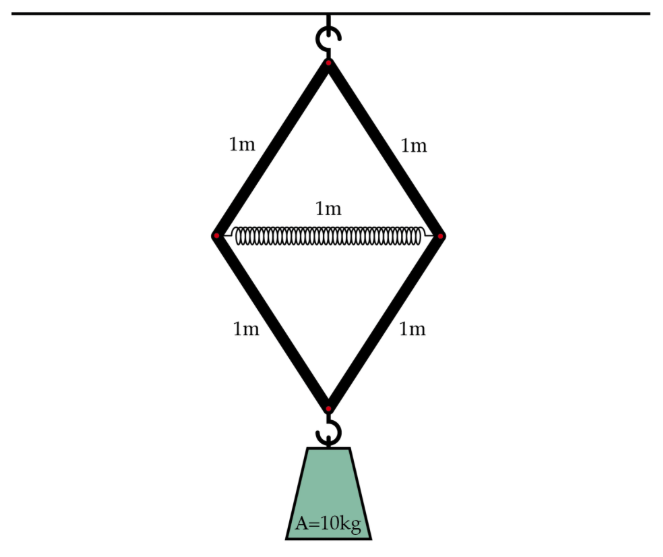# A squeezing springA spring and four bars are hung from the ceiling in the configuration above. Each of the bars can pivot and rotate where it meets the other bar. The spring has a natural length of 1 m and a spring constant of 1000 N/m, while each of the bars has a length of 1 m and is effectively massless. A 10 kg weight is then hooked on to the bottom of this contraption at point A, causing the spring to squeeze. When the system comes to equilibrium, what is the length of the spring in meters?

Details and assumptions

• The acceleration of gravity is $-9.8~m/s^2$.
• The spring doesn't squeeze all that much, so you may assume that the vertical angles on the top and bottom remain at $60+\epsilon$ degrees, where $\epsilon$ is a small angle.
×